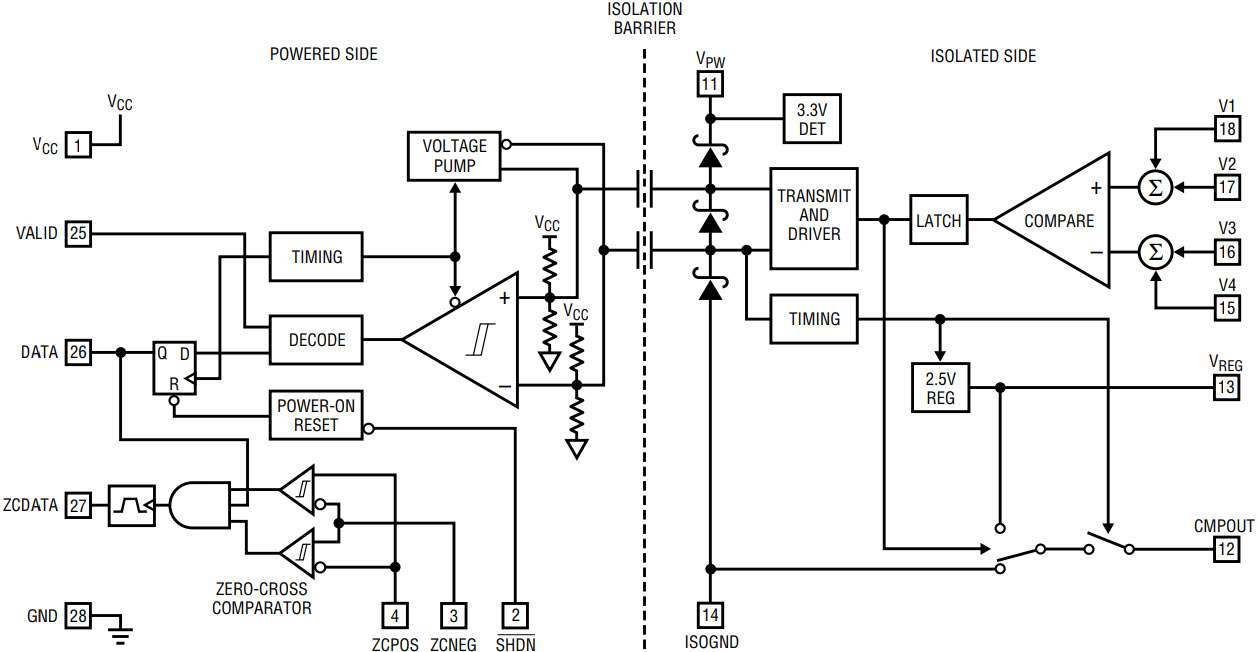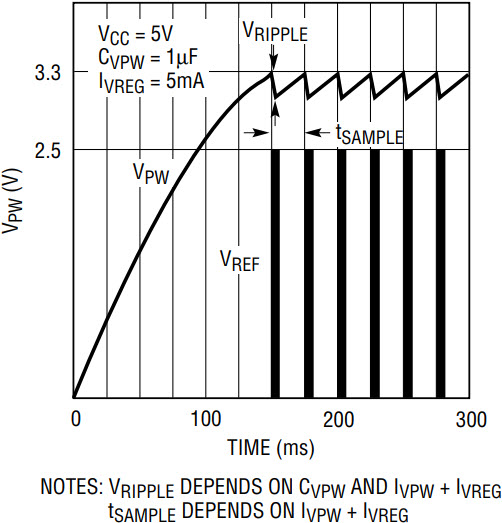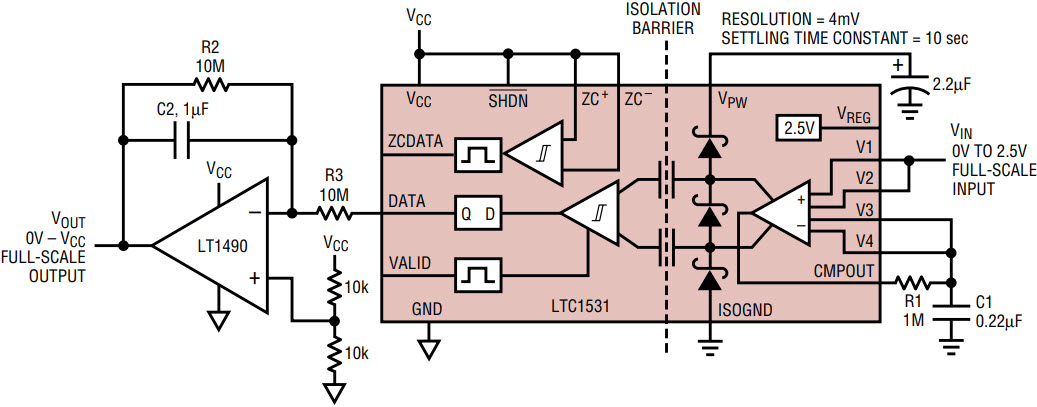# LTC1531 Isolated Comparator

Introduction

The LTC1531 is an isolated, self-powered comparator that receives power and communicates through internal isolation capacitors. The internal isolation capacitors provide 3000VRMS of isolation between the comparator and its output. This allows the part to be used in applications that require high voltage isolated sensing without the need to provide an isolated power source. The isolated side provides a 2.5V pulsed reference output that can deliver 5mA for 100µs using the power stored on the isolated external capacitor. A 4-input, dual-differential comparator samples at the end of the reference pulse and transmits the result back to the nonisolated side. The nonisolated, powered side latches the result of the comparator and provides a zero-cross comparator output for triggering a triac. Typical applications include isolated temperature sensing and control, isolated voltage monitoring and other sensing applications riding on top of high common mode voltages, such as the AC power line.

Basic Operation

The block diagram in Figure 1 shows the basic components of the LTC1531. The nonisolated powered side toggles between pumping AC voltage through the capacitive barrier to the isolated side, where it is rectified and stored on an external capacitor tied to VPW, and listening for a comparison result. When the isolated-side VPW voltage reaches 3.3V, the comparison circuitry is enabled. On the next listen cycle, the 2.5V VREG output pulses on for 100µs, at the end of which a comparison is done, with the result transmitted back to the nonisolated side. If a valid result is received, the DATA output is updated and the VALID output pulses on for 1ms. When the latched DATA output is high, the zero-cross comparator output is enabled for firing a triac whenever the zero-cross comparator inputs pass through 0V.Figure 1. LTC1531 block diagram.

Figure 2 represents a typical VPW start-up sequence, showing VREG output pulses after VPW reaches 3.3V. Thereafter, whenever VPW reaches 3.3V the comparator samples during the next listen period in the power/listen cycle. Figure 2 shows typical sampling with light loading on VREG. Sampling is not uniform but depends on the combination of VPW = 3.3V and the 800Hz power/listen cycle. The comparator samples at a typical rate of 200Hz–300Hz. The actual sampling rate depends on the internal and external loading on the 2.5V VREG output and the charging rate to the external capacitor on VPW. This charging rate, through the internal isolation capacitors to VPW, can be modeled as a 100k source resistance and a 5.5V source with VCC = 5V. Figure 4 shows typical sampling periods for different load currents and supply voltages. The sample rate does not depend on the external storage capacitor, whose value should be chosen to minimize ripple on VPW for different VREG loads. VPW can also be used to power continuous, low current circuits, such as the LT1495 op amp or the LTC1540 comparator, provided that such circuits do not prevent VPW from reaching 3.3V.Figure 2. Typical VPW power-up and VREG samples.Figure 3. Typical VREG and VPW with IVREG = 100µA.Figure 4. Typical average tSAMPLE vs IVREG.

Isolated Comparator

The LTC1531 isolated switched capacitor comparator has four inputs that sum the voltages together to perform the following comparison:

(V1 + V2)/2 > (V3 + V4)/2

By rearranging the equation, for example, a dual differential comparison can be performed:

(V1 – V4) > (V3 – V2) or (V1 – V3) > (V4 – V2)

The comparator inputs have a rail-to-rail input range. They sample once at the end of the 100µs VREG pulse. Their summing nature allows mid-VREG referencing, for example, by connecting V3 to VREG and V4 to ISOGND, which sums together to provide 1.25V for the negative comparator input. In the isolated temperature control application (Figure 5), the comparator is used to compare the voltage across the thermistor to the voltage across R4, with (V1 – V3) > (V4 – V2).Figure 5. Isolated thermistor temperature controller.

The isolated comparator has an isolated output, CMPOUT, which can be used for hysteresis. This output is Hi-Z except when VREG is on; then the output is either 2.5V or 0V, depending on the result of the previous comparison. This output, in combination with the comparator, can be used to create a delta-sigma modulator for transmitting isolated voltage signals across the isolation barrier, as in the isolated voltage sense application (Figure 6).

Applications

The LTC1531 can be used to isolate sensors such as in the isolated thermistor temperature controller in Figure 5. In this circuit, a comparison is made between the voltages across a thermistor and a resistor that is driven by the 2.5V VREG output. As the thermistor resistance rises with temperature, the voltage across the thermistor increases. When it exceeds the voltage across R4, the comparator output becomes zero and the triac control to the heater is turned off. Hysteresis can be added in the temperature control by using CMPOUT and R5. A 10° phase-shifted AC line signal is supplied through R1, R2 and C1 to the zero-cross comparator for firing the triac.

In the overtemperature detect application in Figure 6, an isolated thermocouple is cold junction compensated with the micropower LT1389 reference and the Yellow Springs thermistor. The micropower LT1495 op amp provides gain to give an overall 0°C–200°C temperature range, adjustable by changing the 10M feedback resistor. The isolated comparator is connected to compare at 1.25V or the center of the temperature range. In this case, VTRIP goes high when the temperature exceeds 100°C.

The LTC1531 can use the high impedance nature of CMPOUT as a duty-cycle modulator, as in the isolated voltage sense application in Figure 7. The duty-cycle output of the comparator is smoothed with the LT1490 rail-to-rail op amp to reproduce the voltage at VIN. The output time constant, R2 • C2, should approximately equal the input time constant, 35 • R1 • C1. The factor of 35 results from CMPOUT being on for only 100µs at an average sample rate of 300Hz.Figure 7. Isolated voltage detect.

Conclusion

The LTC1531 is a versatile part for sensing signals that require large isolation voltages. The ability of the LTC1531 to supply power through the isolation barrier simplifies applications; it can be combined with other micropower circuits in a variety of isolated signal conditioning and sensing applications.